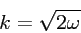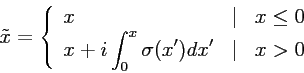### Schrödinger equation - The Perfectly Matched Layer

• Schrödinger equation:
• one-dimensional, in suitably chosen units
•• potential V(x) must be constant outside
• in the following for simplicity V = 0
• plane wave solutions
•with• simple absorbing layer doesn't work
• Basic idea:
• geometry in the following
•• in the boundary x → x + i σ with σ > 0 ⇒ eikx decreases exponentially
• more specific
•• wave function with new coordinate
•• transformed Schrödinger equation
•• has plane wave solutions
•• exponentially decreasing
• Reflections at the boundary:
• disappear only for σ = 0
• remedy: introduce σ(x) with σ(0) = 0, increasing with x
• new coordinate
•• Schrödinger equation becomes
•• result
• waves with exponential decrease
• no reflections